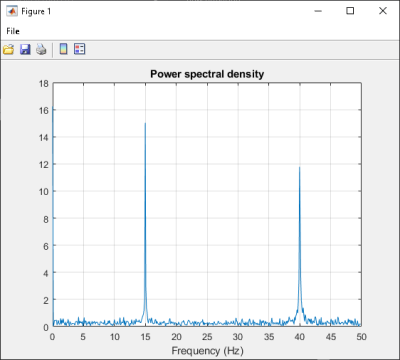Use Multiple Classes in .NET Assembly

This example shows you how to create a .NET assembly that uses multiple classes to analyze a signal and graph the result.

In this example, you perform the following steps:

• Use the MATLAB® Compiler SDK™ product to create an assembly (SpectraComp) containing more than one class.

• Access the component in a C# application (SpectraApp.cs) or a Microsoft® Visual Basic® application (SpectraApp.vb), including use of the MWArray class hierarchy to represent data.

• Build and run the application using the Visual Studio® .NET development environment.

SpectraComp Application

The class SignalAnalyzer performs a fast Fourier transform (FFT) on an input data array. A method of this class, computefft, returns the results of that FFT as two output arrays—an array of frequency points and the power spectral density.

The second class, Plotter, graphs the returned data using the plotfft method. The two methods computefft and plotfft encapsulate MATLAB functions. computefft computes the FFT and power spectral density of the input data and computes a vector of frequency points based on the length of the data entered and the sampling interval. plotfft plots the FFT data and the power spectral density in a MATLAB figure window.

Files

 MATLAB Functions computefft.m plotfft.m MATLAB Function Location matlabroot\toolbox\dotnetbuilder\Examples\VSVersion\NET\SpectraExample\SpectraComp\ C# Code Location matlabroot\toolbox\dotnetbuilder\Examples\VSVersion\NET\SpectraExample\SpectraCSApp\SpectraApp.cs Visual Basic Code Location matlabroot\toolbox\dotnetbuilder\Examples\VSVersion\NET\SpectraExample\SpectraVBApp\SpectraApp.vb

Procedure

1. Copy the following folder that ships with the MATLAB product to your work folder:

matlabroot\toolbox\dotnetbuilder\Examples\VSVersion\NET\SpectraExample

At the MATLAB command prompt, navigate to the new SpectraExample\SpectraComp subfolder in your work folder.

2. Examine the MATLAB functions computefft.m and plotfft.m.

3. Build the .NET component with the Library Compiler app or compiler.build.dotNETAssembly.

Name the library SpectraComp. Map a class named SignalAnalyzer to the function computefft.m, and map a second class named Plotter to the function plotfft.m.

For example, if you are using compiler.build.dotNETAssembly, type:

cmap = containers.Map;
cmap('SignalAnalyzer') = 'computefft.m';
cmap('Plotter') = 'plotfft';
buildResults = compiler.build.dotNETAssembly(cmap, ...
'AssemblyName','SpectraComp');

For more details, see the instructions in Generate .NET Assembly and Build .NET Application.

4. Decide whether you are using C# or Visual Basic to access the component.

• C#

If you are using C#, write source code for a C# application that accesses the component.

The sample application for this example is in SpectraExample\SpectraCSApp\SpectraApp.cs.

The following statement shows how to use the MWArray class library to construct a MWNumericArray that is used as method input to the computefft function.

MWNumericArray data= new MWNumericArray(MWArrayComplexity.Real,
MWNumericType.Double, numSamples);

The following statements create an instance of the class SignalAnalyzer and call the method computefft, requesting 3 outputs.

SignalAnalyzer signalAnalyzer = new SignalAnalyzer();
...
MWArray[] argsOut= signalAnalyzer.computefft(3, data, interval);

• Visual Basic

If you are using Visual Basic, write source code for a Visual Basic application that accesses the component.

The sample application for this example is in SpectraExample\SpectraVBApp\SpectraApp.vb.

The following statements show how to use the MWArray class library to construct the necessary data types:

Dim data As MWNumericArray = New MWNumericArray_
(MWArrayComplexity.Real, MWNumericType.Double, numSamples)
...
Dim resultArray As MWNumericArray = New MWNumericArray_
(MWArrayComplexity.Complex,
MWNumericType.Double, numElements)

The following statements create an instance of the class SignalAnalyzer and call the method computefft, requesting three outputs:

Dim signalAnalyzer As SignalAnalyzer = New SignalAnalyzer
...
Dim argsOut() As MWArray =
signalAnalyzer.computefft(3, data,
MWArray.op_Implicit(interval))

In either case, the SpectraApp program does the following:

• Constructs an input array with values representing a random signal with two sinusoids at 15 and 40 Hz embedded inside of it

• Creates an MWNumericArray array that contains the data

• Instantiates a SignalAnalyzer object

• Calls the computefft method, which computes the FFT, frequency, and the spectral density

• Instantiates a Plotter object

• Calls the plotfft method, which plots the data

• Uses a try/catch block to handle exceptions

5. Open the .NET project file that corresponds to your application language using Visual Studio.

• C#

If you are using C#, the SpectraCSApp folder contains a Visual Studio .NET project file for this example. Open the project in Visual Studio .NET by double-clicking SpectraCSApp.csproj in Windows® Explorer. You can also open it from the desktop by right-clicking SpectraCSApp.csproj and selecting Open Outside MATLAB.

• Visual Basic

If you are using Visual Basic, the SpectraVBApp folder contains a Visual Studio .NET project file for this example. Open the project in Visual Studio .NET by double-clicking SpectraVBApp.vbproj in Windows Explorer. You can also open it from the desktop by right-clicking SpectraVBApp.vbproj and selecting Open Outside MATLAB.

7. Add a reference to the MWArray API.

 If MATLAB is installed on your system matlabroot\toolbox\dotnetbuilder\bin\win64\\MWArray.dll If MATLAB Runtime is installed on your system \toolbox\dotnetbuilder\bin\win64\\MWArray.dll

8. Build and run the SpectraApp application in Visual Studio .NET.

The program displays the following output:

FFT:
1.0e+02 *

4.8646 + 0.0000i
-0.0289 + 0.1080i
-0.0326 + 0.0237i
-0.0141 - 0.0148i
0.0674 - 0.0487i
0.0753 + 0.0669i
0.0275 - 0.0101i
-0.0429 + 0.0472i
0.0803 - 0.1163i
-0.0619 - 0.1072i
0.0565 - 0.0502i
-0.0223 + 0.0587i
-0.0853 - 0.0812i
-0.0662 - 0.0143i
0.0543 - 0.0972i
0.0814 - 0.0463i
-0.0981 - 0.0190i
0.0042 + 0.0083i
-0.0339 + 0.0290i
0.0291 + 0.0036i

Frequency:
0.0000 + 0.0000i
0.0999 + 0.0000i
0.1998 + 0.0000i
0.2997 + 0.0000i
0.3996 + 0.0000i
0.4995 + 0.0000i
0.5994 + 0.0000i
0.6993 + 0.0000i
0.7992 + 0.0000i
0.8991 + 0.0000i
0.9990 + 0.0000i
1.0989 + 0.0000i
1.1988 + 0.0000i
1.2987 + 0.0000i
1.3986 + 0.0000i
1.4985 + 0.0000i
1.5984 + 0.0000i
1.6983 + 0.0000i
1.7982 + 0.0000i
1.8981 + 0.0000i

Power Spectral Density:
15.3755 + 0.0000i
0.3534 + 0.0000i
0.1274 + 0.0000i
0.0646 + 0.0000i
0.2628 + 0.0000i
0.3183 + 0.0000i
0.0925 + 0.0000i
0.2016 + 0.0000i
0.4465 + 0.0000i
0.3912 + 0.0000i
0.2387 + 0.0000i
0.1985 + 0.0000i
0.3723 + 0.0000i
0.2140 + 0.0000i
0.3520 + 0.0000i
0.2960 + 0.0000i
0.3158 + 0.0000i
0.0294 + 0.0000i
0.1411 + 0.0000i
0.0927 + 0.0000i

Plotting Power spectral density, please wait...